Anúncio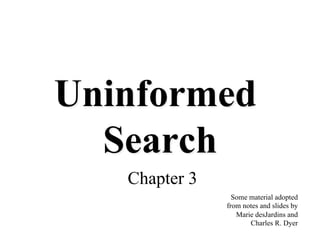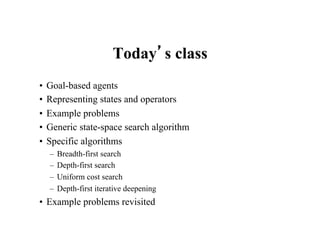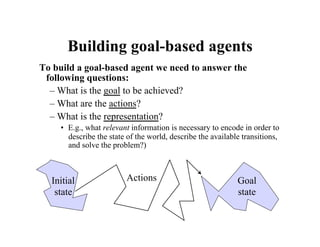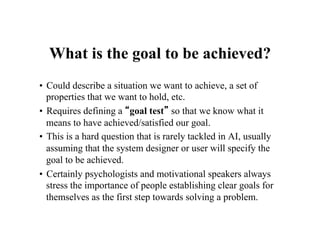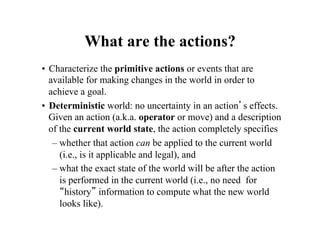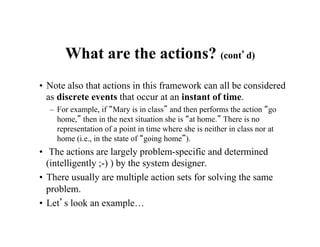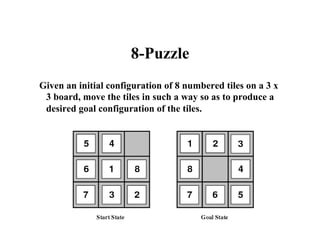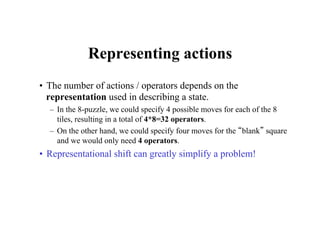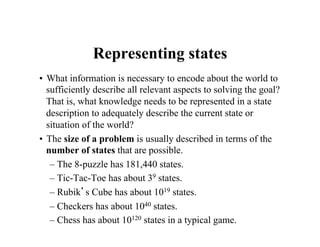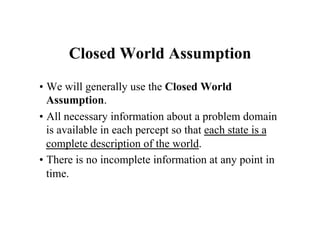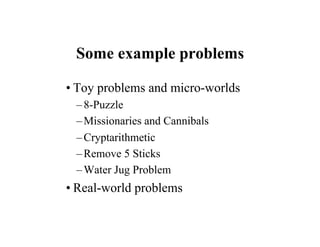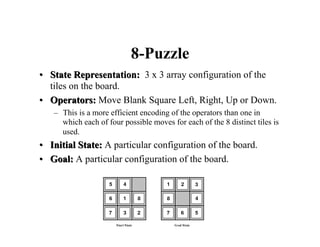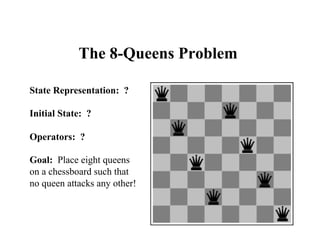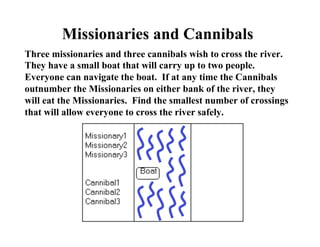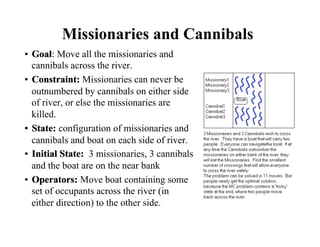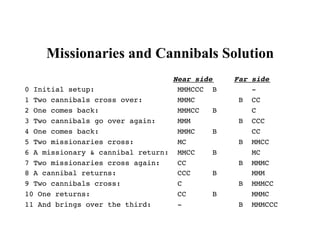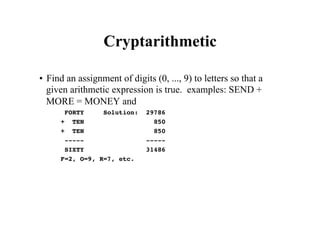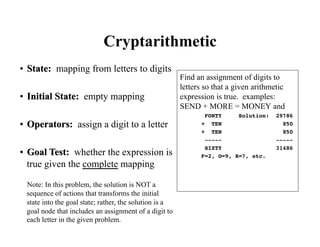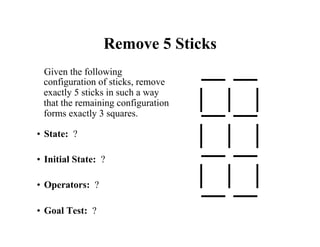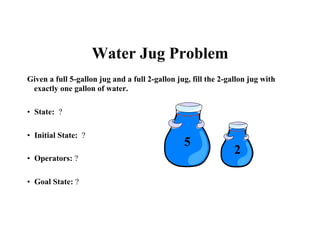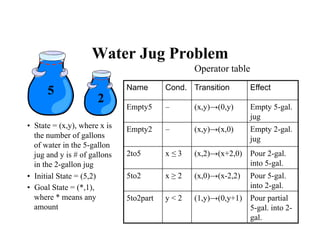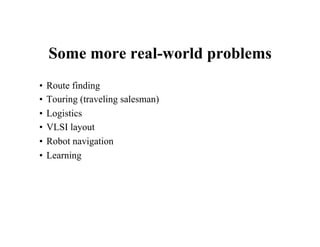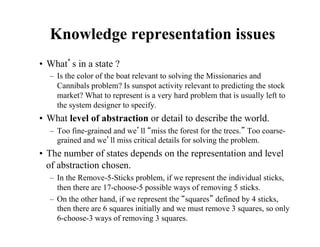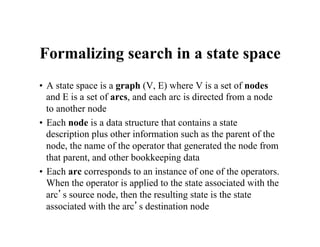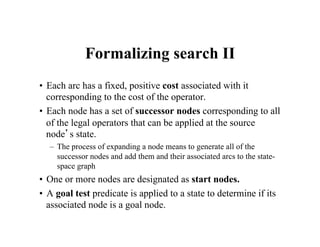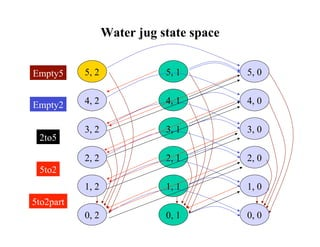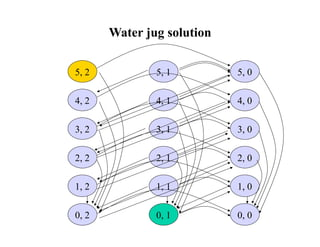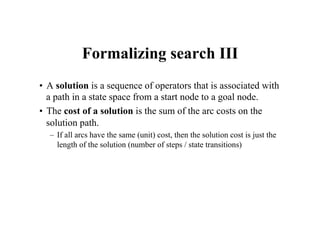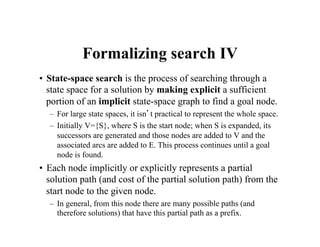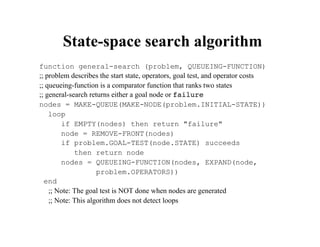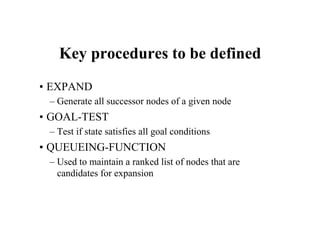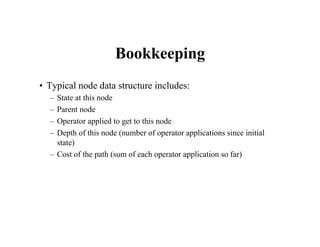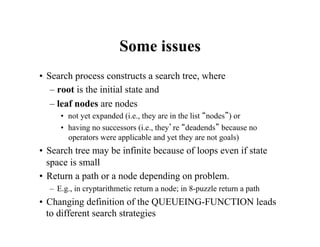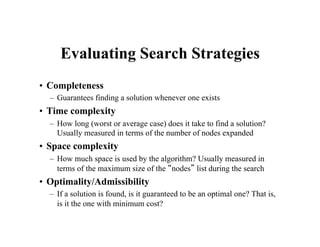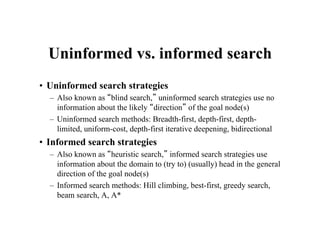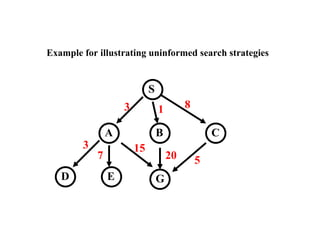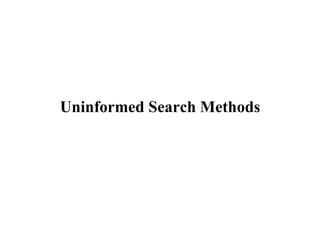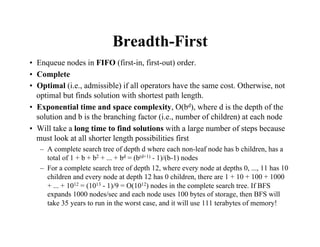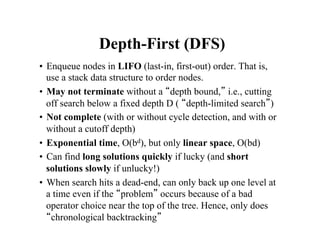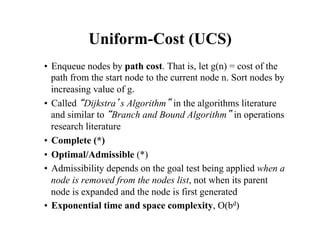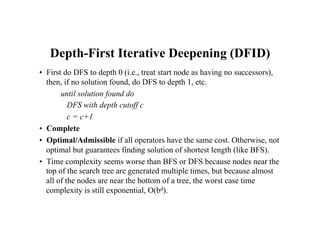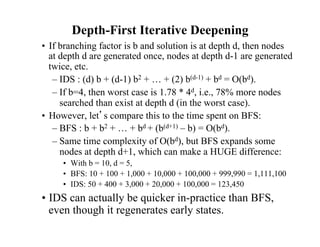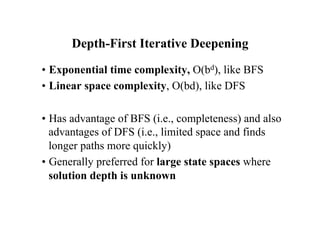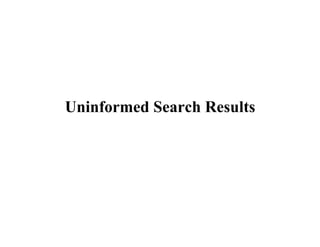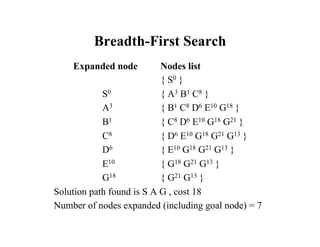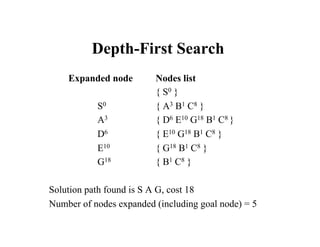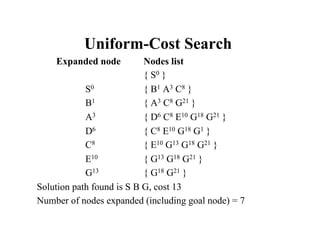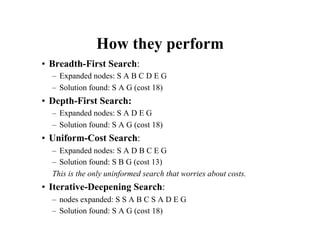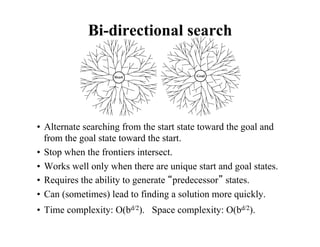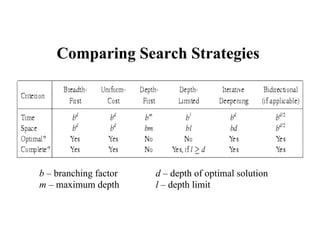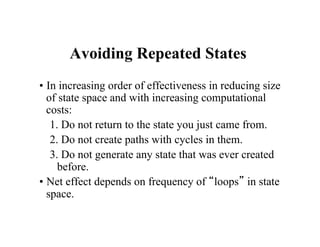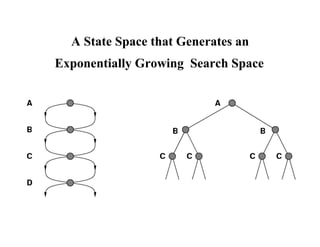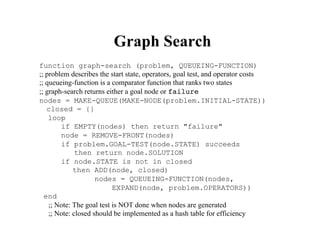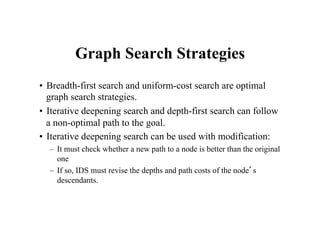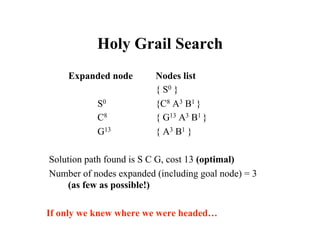1 de 56
Anúncio

### 03_UninformedSearch.pdf

1. Uninformed Search Chapter 3 Some material adopted from notes and slides by Marie desJardins and Charles R. Dyer
2. Todayʼs class • Goal-based agents • Representing states and operators • Example problems • Generic state-space search algorithm • Specific algorithms – Breadth-first search – Depth-first search – Uniform cost search – Depth-first iterative deepening • Example problems revisited
3. Building goal-based agents To build a goal-based agent we need to answer the following questions: – What is the goal to be achieved? – What are the actions? – What is the representation? • E.g., what relevant information is necessary to encode in order to describe the state of the world, describe the available transitions, and solve the problem?) Initial state Goal state Actions
4. What is the goal to be achieved? • Could describe a situation we want to achieve, a set of properties that we want to hold, etc. • Requires defining a “goal test” so that we know what it means to have achieved/satisfied our goal. • This is a hard question that is rarely tackled in AI, usually assuming that the system designer or user will specify the goal to be achieved. • Certainly psychologists and motivational speakers always stress the importance of people establishing clear goals for themselves as the first step towards solving a problem.
5. What are the actions? • Characterize the primitive actions or events that are available for making changes in the world in order to achieve a goal. • Deterministic world: no uncertainty in an actionʼs effects. Given an action (a.k.a. operator or move) and a description of the current world state, the action completely specifies – whether that action can be applied to the current world (i.e., is it applicable and legal), and – what the exact state of the world will be after the action is performed in the current world (i.e., no need for “history” information to compute what the new world looks like).
6. What are the actions? (contʼd) • Note also that actions in this framework can all be considered as discrete events that occur at an instant of time. – For example, if “Mary is in class” and then performs the action “go home,” then in the next situation she is “at home.” There is no representation of a point in time where she is neither in class nor at home (i.e., in the state of “going home”). • The actions are largely problem-specific and determined (intelligently ;-) ) by the system designer. • There usually are multiple action sets for solving the same problem. • Letʼs look an example…
7. 8-Puzzle Given an initial configuration of 8 numbered tiles on a 3 x 3 board, move the tiles in such a way so as to produce a desired goal configuration of the tiles.
8. Representing actions • The number of actions / operators depends on the representation used in describing a state. – In the 8-puzzle, we could specify 4 possible moves for each of the 8 tiles, resulting in a total of 4*8=32 operators. – On the other hand, we could specify four moves for the “blank” square and we would only need 4 operators. • Representational shift can greatly simplify a problem!
9. Representing states • What information is necessary to encode about the world to sufficiently describe all relevant aspects to solving the goal? That is, what knowledge needs to be represented in a state description to adequately describe the current state or situation of the world? • The size of a problem is usually described in terms of the number of states that are possible. – The 8-puzzle has 181,440 states. – Tic-Tac-Toe has about 39 states. – Rubikʼs Cube has about 1019 states. – Checkers has about 1040 states. – Chess has about 10120 states in a typical game.
10. Closed World Assumption • We will generally use the Closed World Assumption. • All necessary information about a problem domain is available in each percept so that each state is a complete description of the world. • There is no incomplete information at any point in time.
11. Some example problems • Toy problems and micro-worlds –8-Puzzle –Missionaries and Cannibals –Cryptarithmetic –Remove 5 Sticks –Water Jug Problem • Real-world problems
12. 8-Puzzle Given an initial configuration of 8 numbered tiles on a 3 x 3 board, move the tiles in such a way so as to produce a desired goal configuration of the tiles.
13. • State Representation: 3 x 3 array configuration of the tiles on the board. • Operators: Move Blank Square Left, Right, Up or Down. – This is a more efficient encoding of the operators than one in which each of four possible moves for each of the 8 distinct tiles is used. • Initial State: A particular configuration of the board. • Goal: A particular configuration of the board. 8-Puzzle • State Representation: 3 x 3 array configuration of the tiles on the board. • Operators: Move Blank Square Left, Right, Up or Down. – This is a more efficient encoding of the operators than one in which each of four possible moves for each of the 8 distinct tiles is used. • Initial State: A particular configuration of the board. • Goal: A particular configuration of the board.
14. The 8-Queens Problem State Representation: ? Initial State: ? Operators: ? Goal: Place eight queens on a chessboard such that no queen attacks any other!
15. Missionaries and Cannibals Three missionaries and three cannibals wish to cross the river. They have a small boat that will carry up to two people. Everyone can navigate the boat. If at any time the Cannibals outnumber the Missionaries on either bank of the river, they will eat the Missionaries. Find the smallest number of crossings that will allow everyone to cross the river safely.
16. • Goal: Move all the missionaries and cannibals across the river. • Constraint: Missionaries can never be outnumbered by cannibals on either side of river, or else the missionaries are killed. • State: configuration of missionaries and cannibals and boat on each side of river. • Initial State: 3 missionaries, 3 cannibals and the boat are on the near bank • Operators: Move boat containing some set of occupants across the river (in either direction) to the other side. Missionaries and Cannibals • Goal: Move all the missionaries and cannibals across the river. • Constraint: Missionaries can never be outnumbered by cannibals on either side of river, or else the missionaries are killed. • State: configuration of missionaries and cannibals and boat on each side of river. • Initial State: 3 missionaries, 3 cannibals and the boat are on the near bank • Operators: Move boat containing some set of occupants across the river (in either direction) to the other side.
17. Missionaries and Cannibals Solution Near side Far side! 0 Initial setup: MMMCCC B -! 1 Two cannibals cross over: MMMC B CC! 2 One comes back: MMMCC B C! 3 Two cannibals go over again: MMM B CCC! 4 One comes back: MMMC B CC! 5 Two missionaries cross: MC B MMCC! 6 A missionary & cannibal return: MMCC B MC! 7 Two missionaries cross again: CC B MMMC! 8 A cannibal returns: CCC B MMM! 9 Two cannibals cross: C B MMMCC! 10 One returns: CC B MMMC! 11 And brings over the third: - B MMMCCC !
18. Cryptarithmetic • Find an assignment of digits (0, ..., 9) to letters so that a given arithmetic expression is true. examples: SEND + MORE = MONEY and FORTY Solution: 29786 ! + TEN 850! + TEN 850! ----- -----! SIXTY 31486! F=2, O=9, R=7, etc.
19. • State: mapping from letters to digits • Initial State: empty mapping • Operators: assign a digit to a letter • Goal Test: whether the expression is true given the complete mapping Cryptarithmetic Find an assignment of digits to letters so that a given arithmetic expression is true. examples: SEND + MORE = MONEY and FORTY Solution: 29786 ! + TEN 850 ! + TEN 850 ! ----- ----- ! SIXTY 31486 ! F=2, O=9, R=7, etc. • State: mapping from letters to digits • Initial State: empty mapping • Operators: assign a digit to a letter • Goal Test: whether the expression is true given the complete mapping Note: In this problem, the solution is NOT a sequence of actions that transforms the initial state into the goal state; rather, the solution is a goal node that includes an assignment of a digit to each letter in the given problem.
20. Remove 5 Sticks Given the following configuration of sticks, remove exactly 5 sticks in such a way that the remaining configuration forms exactly 3 squares. • State: ? • Initial State: ? • Operators: ? • Goal Test: ?
21. Water Jug Problem Given a full 5-gallon jug and a full 2-gallon jug, fill the 2-gallon jug with exactly one gallon of water. • State: ? • Initial State: ? • Operators: ? • Goal State: ? 5 2
22. Water Jug Problem • State = (x,y), where x is the number of gallons of water in the 5-gallon jug and y is # of gallons in the 2-gallon jug • Initial State = (5,2) • Goal State = (*,1), where * means any amount Name Cond. Transition Effect Empty5 – (x,y)→(0,y) Empty 5-gal. jug Empty2 – (x,y)→(x,0) Empty 2-gal. jug 2to5 x ≤ 3 (x,2)→(x+2,0) Pour 2-gal. into 5-gal. 5to2 x ≥ 2 (x,0)→(x-2,2) Pour 5-gal. into 2-gal. 5to2part y < 2 (1,y)→(0,y+1) Pour partial 5-gal. into 2- gal. Operator table 5 2
23. Some more real-world problems • Route finding • Touring (traveling salesman) • Logistics • VLSI layout • Robot navigation • Learning
24. Knowledge representation issues • Whatʼs in a state ? – Is the color of the boat relevant to solving the Missionaries and Cannibals problem? Is sunspot activity relevant to predicting the stock market? What to represent is a very hard problem that is usually left to the system designer to specify. • What level of abstraction or detail to describe the world. – Too fine-grained and weʼll “miss the forest for the trees.” Too coarse- grained and weʼll miss critical details for solving the problem. • The number of states depends on the representation and level of abstraction chosen. – In the Remove-5-Sticks problem, if we represent the individual sticks, then there are 17-choose-5 possible ways of removing 5 sticks. – On the other hand, if we represent the “squares” defined by 4 sticks, then there are 6 squares initially and we must remove 3 squares, so only 6-choose-3 ways of removing 3 squares.
25. Formalizing search in a state space • A state space is a graph (V, E) where V is a set of nodes and E is a set of arcs, and each arc is directed from a node to another node • Each node is a data structure that contains a state description plus other information such as the parent of the node, the name of the operator that generated the node from that parent, and other bookkeeping data • Each arc corresponds to an instance of one of the operators. When the operator is applied to the state associated with the arcʼs source node, then the resulting state is the state associated with the arcʼs destination node
26. Formalizing search II • Each arc has a fixed, positive cost associated with it corresponding to the cost of the operator. • Each node has a set of successor nodes corresponding to all of the legal operators that can be applied at the source nodeʼs state. – The process of expanding a node means to generate all of the successor nodes and add them and their associated arcs to the state- space graph • One or more nodes are designated as start nodes. • A goal test predicate is applied to a state to determine if its associated node is a goal node.
27. 5, 2 3, 2 2, 2 1, 2 4, 2 0, 2 5, 1 3, 1 2, 1 1, 1 4, 1 0, 1 5, 0 3, 0 2, 0 1, 0 4, 0 0, 0 Empty2 Empty5 2to5 5to2 5to2part Water jug state space
28. 5, 2 3, 2 2, 2 1, 2 4, 2 0, 2 5, 1 3, 1 2, 1 1, 1 4, 1 0, 1 5, 0 3, 0 2, 0 1, 0 4, 0 0, 0 Water jug solution
29. Formalizing search III • A solution is a sequence of operators that is associated with a path in a state space from a start node to a goal node. • The cost of a solution is the sum of the arc costs on the solution path. – If all arcs have the same (unit) cost, then the solution cost is just the length of the solution (number of steps / state transitions)
30. Formalizing search IV • State-space search is the process of searching through a state space for a solution by making explicit a sufficient portion of an implicit state-space graph to find a goal node. – For large state spaces, it isnʼt practical to represent the whole space. – Initially V={S}, where S is the start node; when S is expanded, its successors are generated and those nodes are added to V and the associated arcs are added to E. This process continues until a goal node is found. • Each node implicitly or explicitly represents a partial solution path (and cost of the partial solution path) from the start node to the given node. – In general, from this node there are many possible paths (and therefore solutions) that have this partial path as a prefix.
31. State-space search algorithm function general-search (problem, QUEUEING-FUNCTION) ;; problem describes the start state, operators, goal test, and operator costs ;; queueing-function is a comparator function that ranks two states ;; general-search returns either a goal node or failure nodes = MAKE-QUEUE(MAKE-NODE(problem.INITIAL-STATE)) loop if EMPTY(nodes) then return "failure" node = REMOVE-FRONT(nodes) if problem.GOAL-TEST(node.STATE) succeeds then return node nodes = QUEUEING-FUNCTION(nodes, EXPAND(node, problem.OPERATORS)) end ;; Note: The goal test is NOT done when nodes are generated ;; Note: This algorithm does not detect loops
32. Key procedures to be defined • EXPAND – Generate all successor nodes of a given node • GOAL-TEST – Test if state satisfies all goal conditions • QUEUEING-FUNCTION – Used to maintain a ranked list of nodes that are candidates for expansion
33. Bookkeeping • Typical node data structure includes: – State at this node – Parent node – Operator applied to get to this node – Depth of this node (number of operator applications since initial state) – Cost of the path (sum of each operator application so far)
34. Some issues • Search process constructs a search tree, where – root is the initial state and – leaf nodes are nodes • not yet expanded (i.e., they are in the list “nodes”) or • having no successors (i.e., theyʼre “deadends” because no operators were applicable and yet they are not goals) • Search tree may be infinite because of loops even if state space is small • Return a path or a node depending on problem. – E.g., in cryptarithmetic return a node; in 8-puzzle return a path • Changing definition of the QUEUEING-FUNCTION leads to different search strategies
35. Evaluating Search Strategies • Completeness – Guarantees finding a solution whenever one exists • Time complexity – How long (worst or average case) does it take to find a solution? Usually measured in terms of the number of nodes expanded • Space complexity – How much space is used by the algorithm? Usually measured in terms of the maximum size of the “nodes” list during the search • Optimality/Admissibility – If a solution is found, is it guaranteed to be an optimal one? That is, is it the one with minimum cost?
36. Uninformed vs. informed search • Uninformed search strategies – Also known as “blind search,” uninformed search strategies use no information about the likely “direction” of the goal node(s) – Uninformed search methods: Breadth-first, depth-first, depth- limited, uniform-cost, depth-first iterative deepening, bidirectional • Informed search strategies – Also known as “heuristic search,” informed search strategies use information about the domain to (try to) (usually) head in the general direction of the goal node(s) – Informed search methods: Hill climbing, best-first, greedy search, beam search, A, A*
37. Example for illustrating uninformed search strategies S C B A D G E 3 1 8 15 20 5 3 7
38. Uninformed Search Methods
39. Breadth-First • Enqueue nodes in FIFO (first-in, first-out) order. • Complete • Optimal (i.e., admissible) if all operators have the same cost. Otherwise, not optimal but finds solution with shortest path length. • Exponential time and space complexity, O(bd), where d is the depth of the solution and b is the branching factor (i.e., number of children) at each node • Will take a long time to find solutions with a large number of steps because must look at all shorter length possibilities first – A complete search tree of depth d where each non-leaf node has b children, has a total of 1 + b + b2 + ... + bd = (b(d+1) - 1)/(b-1) nodes – For a complete search tree of depth 12, where every node at depths 0, ..., 11 has 10 children and every node at depth 12 has 0 children, there are 1 + 10 + 100 + 1000 + ... + 1012 = (1013 - 1)/9 = O(1012) nodes in the complete search tree. If BFS expands 1000 nodes/sec and each node uses 100 bytes of storage, then BFS will take 35 years to run in the worst case, and it will use 111 terabytes of memory!
40. Depth-First (DFS) • Enqueue nodes in LIFO (last-in, first-out) order. That is, use a stack data structure to order nodes. • May not terminate without a “depth bound,” i.e., cutting off search below a fixed depth D ( “depth-limited search”) • Not complete (with or without cycle detection, and with or without a cutoff depth) • Exponential time, O(bd), but only linear space, O(bd) • Can find long solutions quickly if lucky (and short solutions slowly if unlucky!) • When search hits a dead-end, can only back up one level at a time even if the “problem” occurs because of a bad operator choice near the top of the tree. Hence, only does “chronological backtracking”
41. Uniform-Cost (UCS) • Enqueue nodes by path cost. That is, let g(n) = cost of the path from the start node to the current node n. Sort nodes by increasing value of g. • Called “Dijkstraʼs Algorithm” in the algorithms literature and similar to “Branch and Bound Algorithm” in operations research literature • Complete (*) • Optimal/Admissible (*) • Admissibility depends on the goal test being applied when a node is removed from the nodes list, not when its parent node is expanded and the node is first generated • Exponential time and space complexity, O(bd)
42. Depth-First Iterative Deepening (DFID) • First do DFS to depth 0 (i.e., treat start node as having no successors), then, if no solution found, do DFS to depth 1, etc. until solution found do DFS with depth cutoff c c = c+1 • Complete • Optimal/Admissible if all operators have the same cost. Otherwise, not optimal but guarantees finding solution of shortest length (like BFS). • Time complexity seems worse than BFS or DFS because nodes near the top of the search tree are generated multiple times, but because almost all of the nodes are near the bottom of a tree, the worst case time complexity is still exponential, O(bd).
43. Depth-First Iterative Deepening • If branching factor is b and solution is at depth d, then nodes at depth d are generated once, nodes at depth d-1 are generated twice, etc. – IDS : (d) b + (d-1) b2 + … + (2) b(d-1) + bd = O(bd). – If b=4, then worst case is 1.78 * 4d, i.e., 78% more nodes searched than exist at depth d (in the worst case). • However, letʼs compare this to the time spent on BFS: – BFS : b + b2 + … + bd + (b(d+1) – b) = O(bd). – Same time complexity of O(bd), but BFS expands some nodes at depth d+1, which can make a HUGE difference: • With b = 10, d = 5, • BFS: 10 + 100 + 1,000 + 10,000 + 100,000 + 999,990 = 1,111,100 • IDS: 50 + 400 + 3,000 + 20,000 + 100,000 = 123,450 • IDS can actually be quicker in-practice than BFS, even though it regenerates early states.
44. Depth-First Iterative Deepening • Exponential time complexity, O(bd), like BFS • Linear space complexity, O(bd), like DFS • Has advantage of BFS (i.e., completeness) and also advantages of DFS (i.e., limited space and finds longer paths more quickly) • Generally preferred for large state spaces where solution depth is unknown
45. Uninformed Search Results
46. Breadth-First Search Expanded node Nodes list { S0 } S0 { A3 B1 C8 } A3 { B1 C8 D6 E10 G18 } B1 { C8 D6 E10 G18 G21 } C8 { D6 E10 G18 G21 G13 } D6 { E10 G18 G21 G13 } E10 { G18 G21 G13 } G18 { G21 G13 } Solution path found is S A G , cost 18 Number of nodes expanded (including goal node) = 7
47. Depth-First Search Expanded node Nodes list { S0 } S0 { A3 B1 C8 } A3 { D6 E10 G18 B1 C8 } D6 { E10 G18 B1 C8 } E10 { G18 B1 C8 } G18 { B1 C8 } Solution path found is S A G, cost 18 Number of nodes expanded (including goal node) = 5
48. Uniform-Cost Search Expanded node Nodes list { S0 } S0 { B1 A3 C8 } B1 { A3 C8 G21 } A3 { D6 C8 E10 G18 G21 } D6 { C8 E10 G18 G1 } C8 { E10 G13 G18 G21 } E10 { G13 G18 G21 } G13 { G18 G21 } Solution path found is S B G, cost 13 Number of nodes expanded (including goal node) = 7
49. How they perform • Breadth-First Search: – Expanded nodes: S A B C D E G – Solution found: S A G (cost 18) • Depth-First Search: – Expanded nodes: S A D E G – Solution found: S A G (cost 18) • Uniform-Cost Search: – Expanded nodes: S A D B C E G – Solution found: S B G (cost 13) This is the only uninformed search that worries about costs. • Iterative-Deepening Search: – nodes expanded: S S A B C S A D E G – Solution found: S A G (cost 18)
50. Bi-directional search • Alternate searching from the start state toward the goal and from the goal state toward the start. • Stop when the frontiers intersect. • Works well only when there are unique start and goal states. • Requires the ability to generate “predecessor” states. • Can (sometimes) lead to finding a solution more quickly. • Time complexity: O(bd/2). Space complexity: O(bd/2).
51. Comparing Search Strategies b – branching factor d – depth of optimal solution m – maximum depth l – depth limit
52. Avoiding Repeated States • In increasing order of effectiveness in reducing size of state space and with increasing computational costs: 1. Do not return to the state you just came from. 2. Do not create paths with cycles in them. 3. Do not generate any state that was ever created before. • Net effect depends on frequency of “loops” in state space.
53. A State Space that Generates an Exponentially Growing Search Space
54. Graph Search function graph-search (problem, QUEUEING-FUNCTION) ;; problem describes the start state, operators, goal test, and operator costs ;; queueing-function is a comparator function that ranks two states ;; graph-search returns either a goal node or failure nodes = MAKE-QUEUE(MAKE-NODE(problem.INITIAL-STATE)) closed = {} loop if EMPTY(nodes) then return "failure" node = REMOVE-FRONT(nodes) if problem.GOAL-TEST(node.STATE) succeeds then return node.SOLUTION if node.STATE is not in closed then ADD(node, closed) nodes = QUEUEING-FUNCTION(nodes, EXPAND(node, problem.OPERATORS)) end ;; Note: The goal test is NOT done when nodes are generated ;; Note: closed should be implemented as a hash table for efficiency
55. Graph Search Strategies • Breadth-first search and uniform-cost search are optimal graph search strategies. • Iterative deepening search and depth-first search can follow a non-optimal path to the goal. • Iterative deepening search can be used with modification: – It must check whether a new path to a node is better than the original one – If so, IDS must revise the depths and path costs of the nodeʼs descendants.
56. Holy Grail Search Expanded node Nodes list { S0 } S0 {C8 A3 B1 } C8 { G13 A3 B1 } G13 { A3 B1 } Solution path found is S C G, cost 13 (optimal) Number of nodes expanded (including goal node) = 3 (as few as possible!) If only we knew where we were headed…
Anúncio